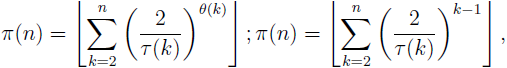# Exact formulae for the prime counting function

Notes on Number Theory and Discrete Mathematics, ISSN 1310-5132
Volume 19, 2013, Number 4, Pages 77—85

## Details

### Authors and affiliations

Mladen Vassilev–Missana5 V. Hugo Str, 1124 Sofia, Bulgaria

### Abstract

In the paper the following explicit formulae for the prime counting function π are proposed and proved:where τ is the number-of-divisors function, θ is either the sum-of-divisors function σ or Dedekind function ψ and ⌊⌋ is the floor function. Also an important general theorem (see Theorem 5) which gives an exact formula (depending on an arbitrary arithmetic function with strictly positive values, satisfying certain condition) for the prime counting function π is formulated and proved. This theorem generalizes all other main results in the paper.

### Keywords

• Prime number
• Composite number
• Arithmetic function

• 11A25
• 11A41

### References

1. Sierpiński, W. Elementary Number Theory, 2nd Edition, North Holland, Amsterdam, 1988.
2. Mitrinović, D., M. Popadić. Inequalities in Number Theory. University of Niš, 1978.

## Cite this paper

Vassilev–Missana, Mladen (2013). Exact formulae for the prime counting function, Notes on Number Theory and Discrete Mathematics, 19(4), 77-85.